# Law of Cosines: Definition and Application

An error occurred trying to load this video.

Try refreshing the page, or contact customer support.

Coming up next: The Double Angle Formula

### You're on a roll. Keep up the good work!

Replay
Your next lesson will play in 10 seconds
• 0:05 Triangles
• 0:49 Law of Cosines
• 2:50 Practice Problem #1
• 4:26 Practice Problem #2
• 5:51 Practice Problem #3
• 7:37 Lesson Summary
Save Save

Want to watch this again later?

Timeline
Autoplay
Autoplay
Speed Speed

#### Recommended Lessons and Courses for You

Lesson Transcript
Instructor
Jeff Calareso

Jeff teaches high school English, math and other subjects. He has a master's degree in writing and literature.

Expert Contributor
Simona Hodis

Simona received her PhD in Applied Mathematics in 2010 and is a college professor teaching undergraduate mathematics courses.

In this lesson, we'll learn how to solve problems involving three sides and one angle in a triangle. The Law of Cosines, a modification of the Pythagorean Theorem, will save the day.

## Triangles

Triangles - they can be troublesome. Love triangles? That's a phrase you never really use to describe a good situation. And the Bermuda triangle? The risk of disappearing into another dimension kind of spoils the otherwise amazing Bermuda vacation.

Fortunately, we have all kinds of great tools to help us with triangles in math problems. Let's say we know two angles and one side length of a triangle. How do we find the missing side? The Law of Sines! That's a/sin A = b/sin B = c/sin C.

The Law of Sines is great for problems involving two angles and two sides. But what about problems involving three sides and one angle? There's another law for that!

## Law of Cosines

It's called the Law of Cosines. Before you think, 'Hey, what's with all these laws? Isn't that kind of restricting? I need to be free, man!' Hold on. This is one of those helpful laws that makes your life easier. It's like a law that requires chocolate chip cookies to be tasty. It's in your best interest, trust me.

Anyway, the law of cosines involves a triangle like this, where our sides are labeled a, b and c. And then we have one angle, like A here.

The law states that a2 = b2 + c2 - 2bc(cosA). So the square of this side equals the sum of the squares of the other two sides minus the cosine of the angle opposite times twice the product of the two other sides.

We can modify the formula to fit whatever angle we have. So it could also be b2 = a2 + c2 - 2ac(cosB) or c2 = a2 + b2 - 2ab(cosC). Just match the corresponding angle and side so they're on opposite sides of the equation.

Does that look a little familiar? Remember the Pythagorean Theorem? a2 + b2 = c2. Notice that the Law of Cosines is the same basic thing, just adding that -2ab(cosC). Why? Because the Pythagorean Theorem only works on right triangles. So this modification accounts for the obliqueness of the triangle. An oblique triangle is just any triangle that isn't a right triangle.

But how can we possibly remember this? Well, the Pythagorean Theorem involves a, b and c, right? The Law of Cosines is the same thing, but with a second a, b and c. So our 2ab(cosC), is our second a, b and c.

## Practice Problem #1

Let's practice. Here's a triangle where we know angle A is 112 degrees. If we know side b is 4 and side c is 15, what is the approximate length of side a?

What's our Law of Cosines? a2 = b2 + c2 - 2bc(cosA). Let's just plug in what we know: a2 = 42 + 152 - 2(4)(15)(cos112). 42 is 16. 152 is 225. Add those to get 241.

The cosine of 112 is about -.37. 2 * 4 is 8. 8 * 15 is 120. 120 * -.37 is -44.4. But since we're supposed to subtract -44.4 from 241, we just add it. That gets us 285.4. So a2 = 285.4. Don't forget that it's a2, not a. That means we need to take the square root of 285.4, which is about 17.

Quick double-check: if side b is 4 and side c is 15, does it make sense that side a is 17? Well, a is clearly the widest angle, so a should be the longest side. Yeah, this seems to make sense. So we're good!

## Practice Problem #2

Let's try another. In this triangle, we know that side a is 12 and side c is 5. We also know that angle B is 33. What is the approximate length of side b?

To unlock this lesson you must be a Study.com Member.

## Law of Cosines- Applications

The law of cosines is a technique applied to a triangle to find the rest of the sides and angles if two sides and the angle between them are given, or all three sides are given.

The two cases are:

SAS (side-angle-side )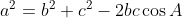and SSS (side-side-side)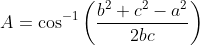### Application

To see how we can practically use the law of cosines, we will find how a flight needs to revise the direction in order to correct a 10-degree error and get to the destination at the same time as scheduled.

In attempting to fly from Houston to San Antonio, a distance of 200 miles, a pilot took a course that was 10 degrees in error. If the aircraft maintains an average speed of 200 mph and if the error is discovered after 15 minutes, we will find out the angle the pilot should turn to head toward San Antonio.

For this, you will do the following.

1) Find the distance traveled by the plane until the error was discovered and draw a triangle to illustrate the path of the plane.

2) Find the third length of the triangle found in 1).

3) Find the angle of correction.

4) Find the new average speed that the pilot should maintain so that the total time of the trip is 60 minutes.

### Solutions

1) The distance traveled by the plane flying at 200 mph for 15 minutes is 5 miles; the triangle with two sides, 200 and 5, and the angle between the two sides of 10 degrees is shown below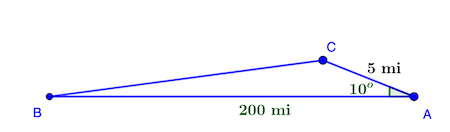2) Applying the law of cosines, we obtain: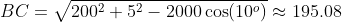3) To find the angle of correction, we can use the law of sines or, again, the law of cosines to obtain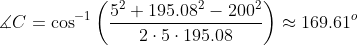4) To travel the total distance in 60 minutes, the pilot needs to travel the BC distance in 45 minutes, so the speed is BC * 60/45 = 260.1 mph.

### Register to view this lesson

Are you a student or a teacher?

#### See for yourself why 30 million people use Study.com

##### Become a Study.com member and start learning now.
Back
What teachers are saying about Study.com

### Earning College Credit

Did you know… We have over 200 college courses that prepare you to earn credit by exam that is accepted by over 1,500 colleges and universities. You can test out of the first two years of college and save thousands off your degree. Anyone can earn credit-by-exam regardless of age or education level.